## Example Questions

← Previous 1

### Example Question #1 : How To Find The Volume Of A Sphere

A cube with sides of 4” each contains a floating sphere with a radius of 1”.  What is the volume of the space outside of the sphere, within the cube?

11.813 in3

64 in3

4.187 in3

11.813 in3

59.813 in3

59.813 in3

Explanation:

Volume of Cube = side3 = (4”)3 = 64 in3

Volume of Sphere = (4/3) * π * r3 = (4/3) * π * 13 = (4/3) * π * 13 = (4/3) * π = 4.187 in3

Difference = Volume of Cube – Volume of Sphere = 64 – 4.187 = 59.813 in3

### Example Question #2 : Spheres

If a sphere's diameter is doubled, by what factor is its volume increased?

4

8

16

3

2

8

Explanation:

The formula for the volume of a sphere is 4πr3/3. Because this formula is in terms of the radius, it would be easier for us to determine how the change in the radius affects the volume. Since we are told the diameter is doubled, we need to first determine how the change in the diameter affects the change in radius.

Let us call the sphere's original diameter d and its original radius r. We know that d = 2r.

Let's call the final diameter of the sphere D. Because the diameter is doubled, we know that D = 2d. We can substitute the value of d and obtain D = 2(2r) = 4r.

Let's call the final radius of the sphere R. We know that D = 2R, so we can now substituate this into the previous equation and write 2R = 4r. If we simplify this, we see that R = 2r. This means that the final radius is twice as large as the initial radius.

The initial volume of the sphere is 4πr3/3. The final volume of the sphere is 4πR3/3. Because R = 2r, we can substitute the value of R to obtain 4π(2r)3/3. When we simplify this, we get 4π(8r3)/3 = 32πr3/3.

In order to determine the factor by which the volume has increased, we need to find the ratio of the final volume to the initial volume.

32πr3/3 divided by 4πr3/3 = 8

The volume has increased by a factor of 8.

### Example Question #3 : Spheres

A sphere increases in volume by a factor of 8. By what factor does the radius change?

8

4

10

6

2

2

Explanation:

Volume of a sphere is 4Πr3/3. Setting that equation equal to the original volume, the new volume is given as 8*4Πr3/3, which can be rewritten as 4Π8r3/3, and can be added to the radius value by 4Π(2r)3/3 since 8 si the cube of 2. This means the radius goes up by a factor of 2

### Example Question #4 : Spheres

A foam ball has a volume of 2 units and has a diameter of x. If a second foam ball has a radius of 2x, what is its volume?

16 units

2 units

8 units

128 units

4 units

128 units

Explanation:

Careful not to mix up radius and diameter. First, we need to identify that the second ball has a radius that is 4 times as large as the first ball.  The radius of the first ball is (1/2)x and the radius of the second ball is 2x. The volume of the second ball will be 43, or 64 times bigger than the first ball.  So the second ball has a volume of 2 * 64 = 128.

### Example Question #1941 : Hspt Mathematics

A cross-section is made at the center of a sphere.  The area of this cross-section is 225π square units.  How many cubic units is the total volume of the sphere?

4500π

3375π

1687.5π

13500π

4500π

Explanation:

The solution to this is simple, though just take it step-by-step.  First find the radius of the circular cross-section.  This will give us the radius of the sphere (since this cross-section is at the center of the sphere).  If the cross-section has an area of 225π, we know its area is defined by:

A = 225π = πr2

Solving for r, we get r = 15.

From here, we merely need to use our formula for the volume of a sphere:

V = (4 / 3)πr3

For our data this is: (4 / 3)π * 153 = 4π * 152 * 5 = 4500π

### Example Question #1942 : Hspt Mathematics

At x = 3, the line y = 4x + 12 intersects the surface of a sphere that passes through the xy-plane. The sphere is centered at the point at which the line passes through the x-axis. What is the volume, in cubic units, of the sphere?

4896π√(17)

816π√(11)

4896π

2040π√(7)

4896π√(17)

Explanation:

We need to ascertain two values: The center point and the point of intersection with the surface.  Let's do that first:

The center is defined by the x-intercept. To find that, set the line equation equal to 0 (= 0 at the x-intercept):

0 = 4x + 12; 4x = –12; x = –3; Therefore, the center is at (–3,0)

Next, we need to find the point at which the line intersects with the sphere's surface. To do this, solve for the point with x-coordinate at 3:

y = 4 * 3 + 12; y = 12 + 12; y = 24; therefore, the point of intersection is at (3,24)

Reviewing our data so far, this means that the radius of the sphere runs from the center, (–3,0), to the edge, (3,24).  If we find the distance between these two points, we can ascertain the length of the radius.  From that, we will be able to calculate the volume of the sphere.

The distance between these two points is defined by the distance formula:

d = √( (x1 – x0)2 + (y1 – y0))

For our data, that is:

√( (3 + 3)2 + (24 – 0)) = √( 62 + 24)  = √(36 + 576) = √612 = √(2 * 2 * 3 * 3 * 17) = 6√(17)

Now, the volume of a sphere is defined by: V = (4/3)πr3

For our data, that would be:  (4/3)π * (6√(17))3 = (4/3) * 63 * 17√(17) * π = 4 * 2 * 62 * 17√(17) * π = 4896π√(17)

### Example Question #1 : Solid Geometry

The surface area of a sphere is 676π in2.  How many cubic inches is the volume of the same sphere?

8788π

(8788π)/3

2028π

(2028π)/3

(2197π)/3

(8788π)/3

Explanation:

To begin, we must solve for the radius of our sphere.  To do this, recall the equation for the surface area of a sphere: A = 4πr2

For our data, that is: 676π = 4πr2; 169 = r2; r = 13

From this, it is easy to solve for the volume of the sphere.  Recall the equation:

V = (4/3)πr3

For our data, this is: V = (4/3)π * 133 = (4π * 2197)/3 = (8788π)/3

### Example Question #1944 : Hspt Mathematics

What is the difference between the volume and surface area of a sphere with a radius of 6?

133π

144π

216π

720π

288π

144π

Explanation:

Surface Area = 4πr2 = 4 * π * 62 = 144π

Volume = 4πr3/3 = 4 * π * 63 / 3 = 288π

Volume – Surface Area = 288π – 144π = 144π

### Example Question #1945 : Hspt Mathematics

A sphere is perfectly contained within a cube that has a volume of 216 units. What is the volume of the sphere?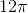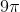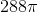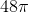Explanation:

To begin, we must determine the dimensions of the cube. This is done by solving the simple equation: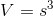We know the volume is 216, allowing us to solve for the length of a side of the cube.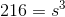Taking the cube root of both sides, we get s = 6.

The diameter of the sphere will be equal to side of the cube, since the question states that the sphere is perfectly contained. The diameter of the sphere will be 6, and the radius will be 3.

We can plug this into the equation for volume of a sphere: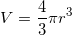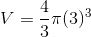We can cancel out the 3 in the denominator.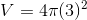Simplify.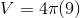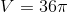### Example Question #2 : How To Find The Volume Of A Sphere

The surface area of a sphere is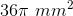. Find the volume of the sphere in cubic millimeters.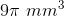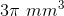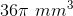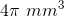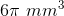Explanation: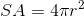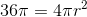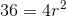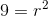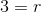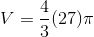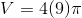← Previous 1